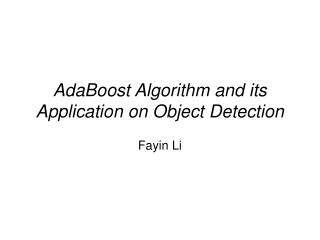Download PresentationAdaBoost Algorithm and its Application on Object Detection

# AdaBoost Algorithm and its Application on Object Detection

Download Presentation## AdaBoost Algorithm and its Application on Object Detection

- - - - - - - - - - - - - - - - - - - - - - - - - - - E N D - - - - - - - - - - - - - - - - - - - - - - - - - - -
##### Presentation Transcript

1. Motivation and Outline • Object detection can be considered as a classification problem (Object / Non-Object) • Rowley, Baluja & Kanade use a two-layer neural network to detect faces. • Sung and Poggio use SVM to detect face and Pedestrian. • Too many features… • How to select features efficiently?? • Adaboost Algorithm • Its application on face / pedestrian detection

2. Adaboost Algorithm • Combine the results of multiple “weak” classifier into a single “strong” classifier: • Reusing or selecting data • Adaptive re-weighting the samples and combing • Given: training data (x1,y1),…,(xm, ym), where xiX, yi Y={-1,+1} • For t = 1,…,T: • Train Weak Classifier ht: X  Y on the training data • Modify the training set somehow • The final hypothesis H(x) is some combination of all weak hypothesis H(x) = f(h(x)) • Question: How to modify the training set and how to combine?? (Bagging: random selection and voting for final hypothesis)

3. Adaboost Algorithm • Two main modifications in Boosing • Instead of a random sample of the training data, use a weighted sample to focus on most difficult examples • Instead of combining classifiers with equal vote, use a weighted vote

4. Weak Classifier 1 Weights Increased Weak Classifier 2 Weak classifier 3 Final classifier is linear combination of weak classifiers Updating the weight of examples

6. How to Choose  • We can show that classification error is minimized by minimizing Zt • If the sample xi is classified wrong, • Thus minimizing Zt will minimize this error bound • Therefore we should choose t to minimize Zt • We should modify the “weak classifier” to minimize Zt instead of the squared error

7. Compute  analytically • If we restrict ,then if the example is classified correctly; otherwise • Let ,find  by setting and we can get • Then the weight updating rules will be and normalize the weights. If the example is classified correctly, the weight will be decreased. And the weights are increased for examples which are classified wrong. • In practice, we can define different loss function for the weak hypothesis. Freund and Schapire define a pseudo-loss function.

8. A variantAdaboost Algorithm (Paul Viola) • If we restrict , similar to above, we will minimize the function • If we denote be the weight error of false negative, be the weight error of false positive, be total weight of positive examples and be total weight of negative examples, we can get  and  minimizing Z : • And the weights are updated similar to above • The decision function

9. Feature Selection • For each round of boosting: • Evaluate each rectangle filter on each example • Sort examples by filter values • Select best threshold for each filter (min Z) • Select best filter/threshold (= Feature) • Reweight examples • M filters, T thresholds, N examples, L learning time • O( MT L(MTN) ) Naïve Wrapper Method • O( MN ) Adaboost feature selector

10. Discussion on Adaboost • Learning Efficient: In each round the entire dependence on previously selected features is efficiently and compactly encoded using the example weighted, which evaluate the weak classifier in constant time • The error of strong classifier approaches zeros exponentially in the number of rounds • Adaboost achieves large margins rapidly • No parameters to tune (except T) • Weak classifier: decision tree, nearest neighbor, simple rule of thumb,…. (Paul Viola restricted weak hypothesis on a single feature) • The week classifier should not be too strong • The hypothesis should not be too complex (Low generalization ability with complex hypothesis)

11. Boosting Cascading • Similar to a decision tree • Smaller and more efficient boosted classifier can be learned to reject many negative and detect almost all positive. • Simpler classifiers to reject major negative and more complex classifier to achieve low false positive rates • The number of classifier stages, the number of features of each stage and the threshold of each stage • The false positive rate of cascade • The total detection rate

12. % False Pos 0 50 vs false neg determined by 50 100 % Detection T T T T IMAGE SUB-WINDOW Classifier 2 Classifier 3 Object Classifier 1 F F F F NON-Object NON-Object NON- NON-Object Cascading Classifiers for Object Detection • Given a nested set of classifier hypothesis classes • Computational Risk Minimization. Each classifier has 100% detection rate and the cascading reduces the false positive rate

13. Some Results